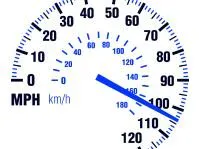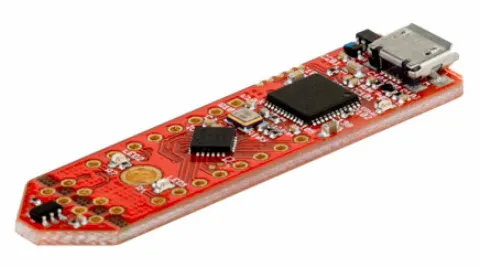Created August 21, 2018 © GPL3+

# Speed Sensor

This is a small magnetic sensor that reports back the rpm so that the speed can be calculated.

BeginnerFull instructions provided30 minutes26## Things used in this project

### Hardware componentsInfineon 3D Magnetic Sensor 2Go
×1

### Software apps and online servicesArduino IDEThe Processing Foundation Processing

## Code

### magnetic sensor code

Arduino
This code will determine the rpm of the magnetic passing by the sensor.

Note: there seems to be an issue where if the magnet is passing by slowly it produces false rpm values.
```#include <Tle493d.h>
//redefinition of abs,  there seems to be an issue where abs will return a negitive number,  this will only return a positive number.
#define absX(x) ((x)<0?-(x):(x))

Tle493d Tle493dMagnetic3DSensor = Tle493d();
long time = micros();

int t;
int cur_t;
int value = 0;

void setup() {
Serial.begin(9600);
while (!Serial);
Tle493dMagnetic3DSensor.begin();
Tle493dMagnetic3DSensor.enableTemp();
}

void loop() {

Tle493dMagnetic3DSensor.updateData();
int value = absX((int)Tle493dMagnetic3DSensor.getX());

if (value > 0 ) {
cur_t = micros();
Serial.println(1000000 * 60 / (cur_t - t));
t = micros();
while (absX((int)Tle493dMagnetic3DSensor.getNorm()) > 0){
Tle493dMagnetic3DSensor.updateData();
}
delay(1); //just needed to help with stabilizing the sensor readings.
}
}
```

### Processing Code visual speedometer

Processing
This code will take the rpm from the sensor and convert it to km/h based on a 66 cm diameter tire.
```import processing.serial.*; //Import Serial library

Serial port; //Serial port name

PrintWriter output;  //Text file for writing sensor data
int cx, cy;
float circleDiametre;
int lf = 10;
float valor;//Sensor value
String speed;

void setup() {
size(640, 360);
stroke(255);

int radius = min(width,height) / 2;
cx = width / 2;
cy = height / 2;

println(Serial.list()); //Serail port list
port = new Serial(this, Serial.list(), 9600);

output = createWriter("speed_data.txt"); //Create Text file . By default in same sketch folder

}

void draw() {
background(20);

//Getting data sensor from Arduino
if(port.available() > 0) // si hay algún dato disponible en el puerto
{
speed = "";
println(speed);
if (speed != null){
// valor = rpm * pi * diameter in cm * cm/min --> km/h conversion value
valor = abs(Integer.parseInt(trim(speed))) * 3.14 * 66 * 0.000599999999999999;
println(speed);
}
}
//Data visualization
text((int)valor,0.8 * width,height/2);
text("km/h", 0.8 * width,(height + 50 )/2);

//Writing data in the text file
output.print(valor + "km/h");
output.println("");

fill(55);
noStroke();
ellipse(cx,cy,circleDiametre,circleDiametre);//draw the speedometer background

// Angles for sin() and cos() start at 3 o'clock;
// subtract HALF_PI to make them start at the top
float s = map(valor, 0, 25, 0, HALF_PI) + 3 * QUARTER_PI ;

// Draw the handle
stroke(167,20,20);
strokeWeight(4);
line(cx, cy, cx + cos(s) * speedRadius, cy + sin(s) * speedRadius);
delay(100);
// Draw the speed ticks
stroke(255);
strokeWeight(8);
beginShape(POINTS);
for (int a = 135; a < 410; a+=20) {
float x = cx + cos(angle) * speedRadius;
float y = cy + sin(angle) * speedRadius;
vertex(x, y);
}

}
```

## Credits

### Dana Mah

11 projects • 19 followers
I'm a hobbyist interested in microcontroller solutions to simple problems.